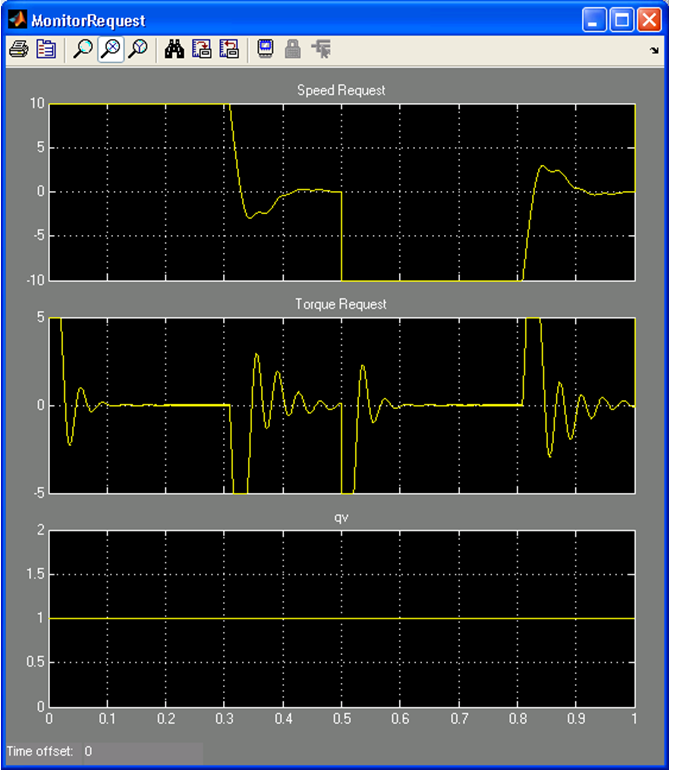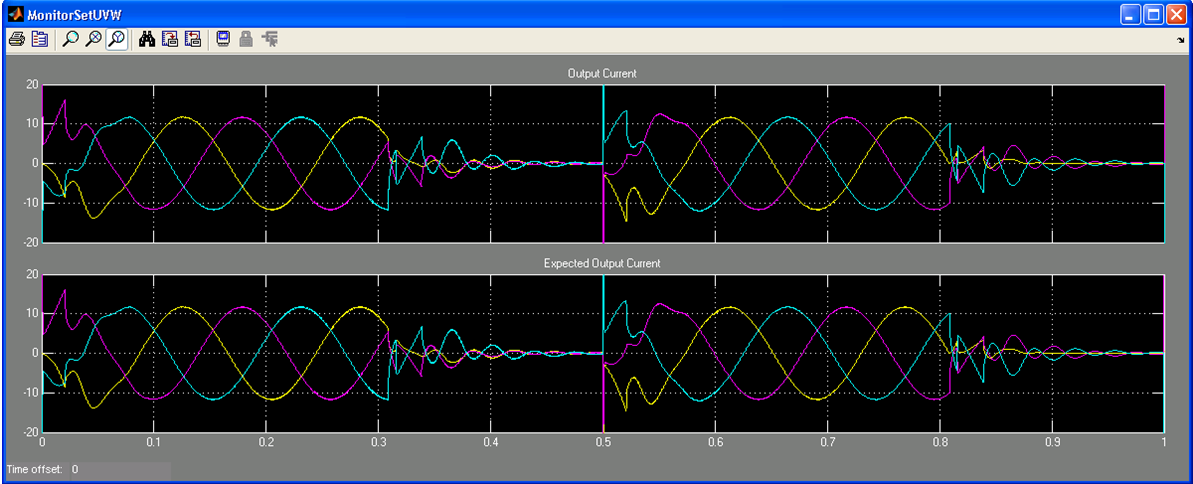ID 683337
Date 5/01/2023
Public

Give Feedback

## 7.6.1. Position, Speed, and Current Control for AC Motors

This design example implements a field-oriented control (FOC) algorithm for AC motors such as permanent magnet synchronous machines (PMSM). Industrial servo motors, where the precise control of torque is important, commonly use these algorithms. This design example includes position and speed control, which allow the control of rotor speed and angle.

Note: Intel has not tested this design on hardware and Intel does not provide a model of a motor.

The model file is psc_ctrl.mdl. Also, an equivalent fixed-point design, psc_ctrl_fixed.mdl, exists. To change the precision this design uses, refer to the setup_position_speed_current_controller_fixed.m script.

### Functional Description

An encoder measures the rotor position in the motor, which the FPGA then reads. An analog-to-digital converter (ADC) measures current feedback, which the FPGA then reads.

Figure 56. AC Motor Control System Block Diagram

Each of the FOC, speed, and position feedback loops use a simple PI controller to reduce the steady state error to zero. In a real-world PI controller, you may also need to consider integrator windup and tune the PI gains appropriately. The feedback loops for the integral portion of the PI controllers are internal to the design.

The example assumes you sample the inputs at a rate of 100 kHz and the FPGA clock rate is 100 MHz (suitable for Cyclone IV devices). ALU folding reduces the resource usage by sharing operators such as adders, multipliers, cosine. The folding factor is set to 100 to allow each operator to be timeshared up 100 times, which gives an input sample rate of 1 Msps, but as the real input sample rate is 100 ksps, only one out of every ten input timeslots are used. DSP Builder identifies the used timeslots when valid_in is 1. Use valid_in to enable the latcher in the PI controller, which stores data for use in the next valid timeslot. The valid_out signal indicates when the ChannelOut block has valid output data. You can calculate nine additional channels on the samedesign without incurring extra latency (or extra FPGA resources).

You should adjust the folding factor to see the effect it has on hardware resources and latency. To adjust, change the Sample rate (MHz) parameter in the ChannelIn and ChannelOut blocks of the design either directly or change the FoldingFactor parameter in the setup script. For example, a clock frequency of 100 MHz and sample rate of 10 MHz gives a folding factor of 10. Disabling folding, or setting the factor to 1, results in no resource sharing and minimal latency. Generally, you should not set the folding factor greater than the number of shareable operators, that is, for 24 adders and 50 multipliers, use a maximum folding factor 50.

Note: The testbench does not support simulations if you adjust the folding factor.

The control algorithm, with the FOC, position, speed, control loops, vary the desired position across time. The three control loops are parameterized with minimum and maximum limits, and Pl values. These values are not optimized and are for demonstrations only.

### Resource Usage

Table 15.  Position, Speed, and Current Control for AC Motors Design Example Resource Usage
Folding Factor Add and Sub Blocks Mult Blocks Cos Blocks Latency
No folding 22 22 4 170
>22 1 1 1 279

The example uses floating-point arithmetic that automatically avoids arithmetic overflow, but you can implement it in a fixed-point design and tune individual accuracies while manually avoiding overflows.

### Hardware Generation

When hardware generation is disabled, the Simulink system simulates the design at the external sample rate of 100 kHz, so that it outputs a new value once every 100 kHz. When hardware generation is enabled, the design simulates at the FPGA clock rate (100 MHz), which represents real-life latency clock delays, but it only outputs a new value every 100 kHz. This mode slows the system simulation speed greatly as the model is evaluated 1,000 times for every output. The setup script for the design example automatically detects whether hardware generation is enabled and sets the sample rates accordingly. The example is configured with hardware generation disabled, which allows fast simulations. When you enable hardware generation, set a very small simulation time (for example 0.0001 s) as simulation may be very slow.
Figure 57. Input Position RequestAt 0 s, a position of 3 is requested and then at 0.5 s a position of 0 is requested. Also shows the actual position and motor feedback currentsFigure 58. Output Response for Speed and TorqueThe maximum speed request saturates at 10 and the torque request saturates at 5 as set by parameters of the model. Also, some oscillation exists on the speed and torque requests because of nonoptimal settings for the PI controller causing an under-damped response.Figure 59. Output CurrentFrom 0 to 0.1, the motor is accelerating; 0.1 to 0.3, it is at a constant speed; 0.3 to 0.5, it is decelerating to stop. From 0.5 to 0.6, the motor accelerates in the opposite direction; from 0.6 to 0.8, it is at a constant speed; from 0.8 to 1, it is decelerating to stop.